# SAT II Chemistry : Solutions and States of Matter

## Example Questions

### Example Question #1 : Gas Laws

Suppose that an ideal gas atand 2.0 atm has a volume of 11.2 cubic meters. Which of the following expressions represents the volume of the gas, in cubic meters, if the temperature were increased byand the pressure decreased to 1.5 atm?Explanation:

First of all, the unit degrees Celsius should be converted to Kelvins by adding 273. This means that the temperature increases from 273 K to 323 K.

Next, the combined gas law can be used.Plugging in the known values givesand solving for the volume givesRearranging shows this is clearly equivalent to the correct answer,.

### Example Question #1 : Solutions And States Of Matter

How much faster is the rate of effusion of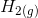than the rate of effusion of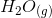?Explanation:

By Graham's Law,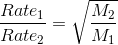. The molar mass of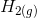is 2 g/mol and the molar mass ofis 18 g/mol. Thus,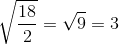### Example Question #2 : Solutions And States Of Matter

30 mL of 1.0 M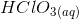solution is diluted with water to a volume of 3 L. What is the new concentration of the solution?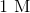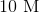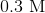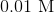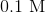Recall that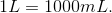Thus, the volume of the solution increased from 30 mL to 3000 mL, which is a factor of 100. Thus, the new concentration must be 100 times less than the original concentration, and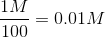.